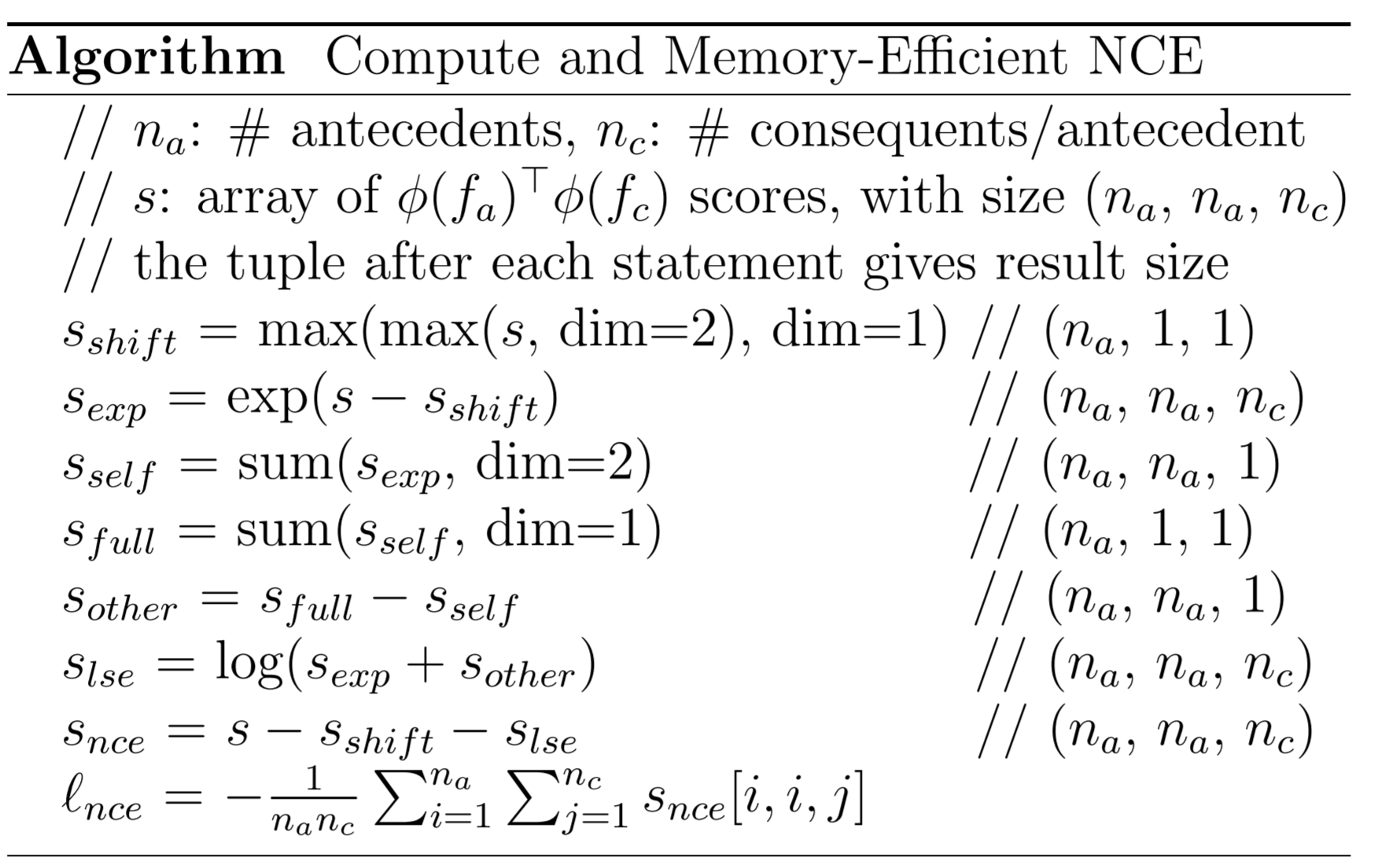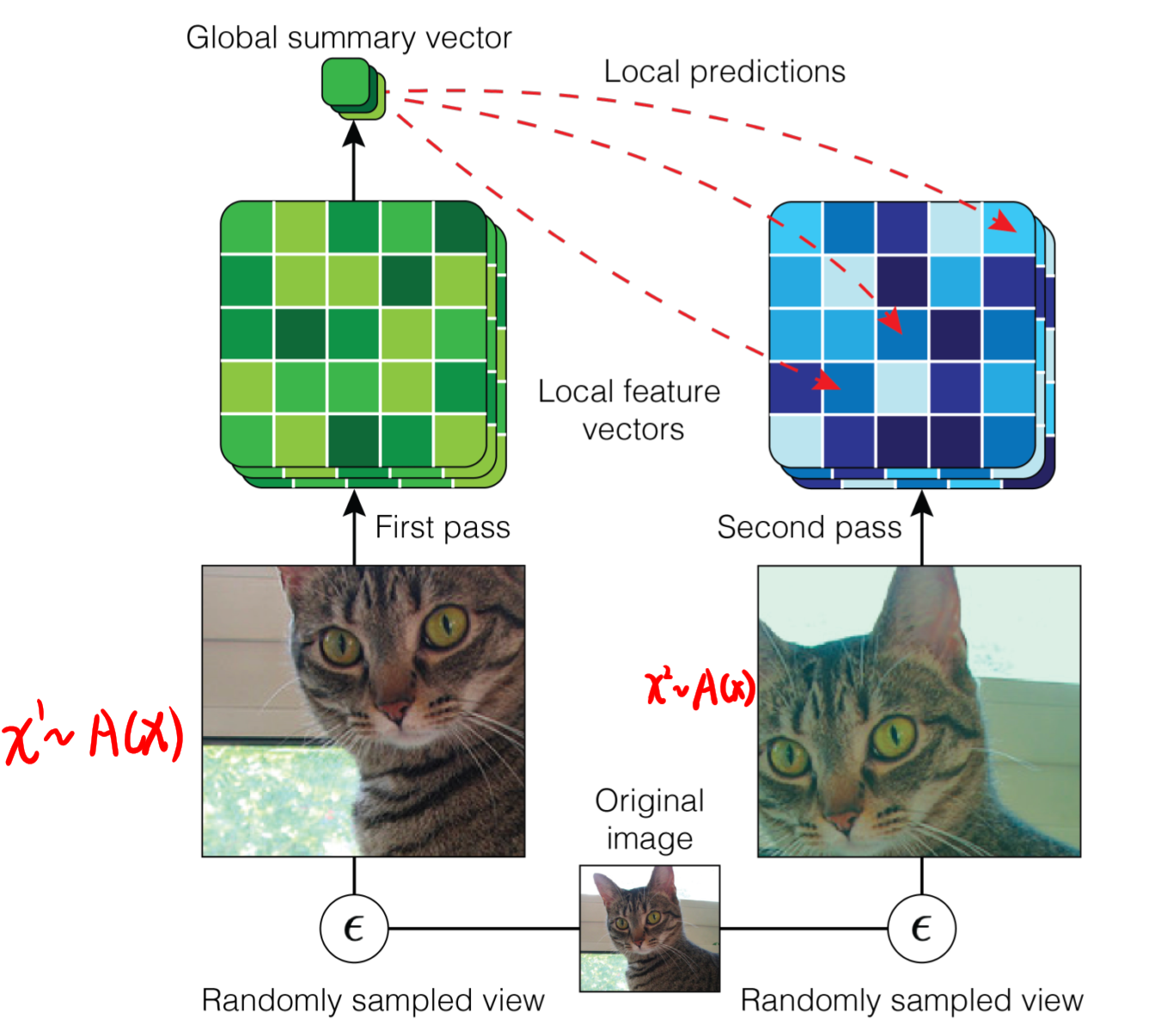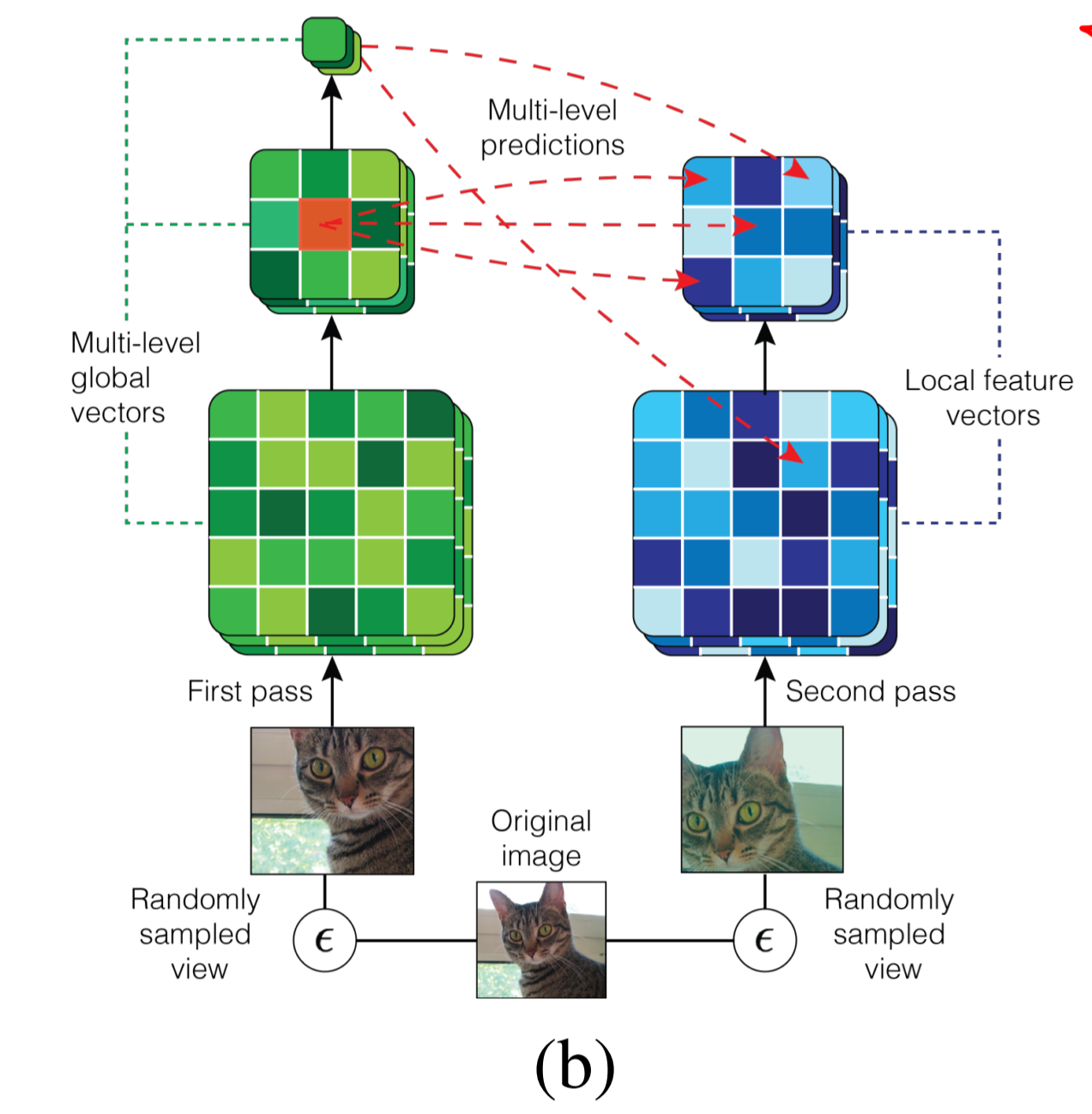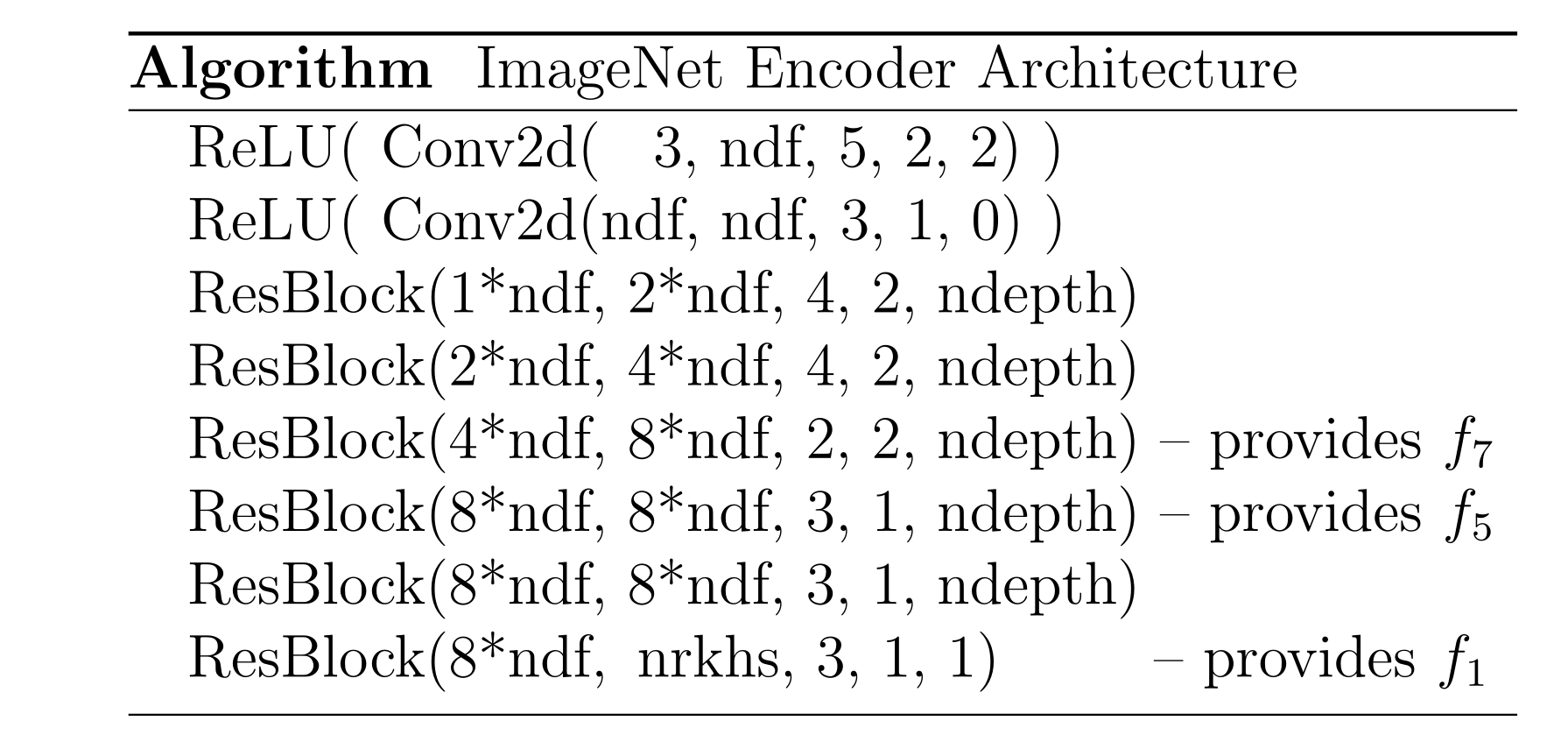# 【论文笔记——AMDIM】Learning Representations by Maximizing Mutual Information Across Views

arxiv：https://arxiv.org/abs/1906.00910
code：https://github.com/Philip-Bachman/amdim-public

# 1 Introduction

• 从图像的独立增强副本(independently-augmented copies)中提取特征，而不是原始图像。
• 最大化多尺度特征(mutiple feature scales)间的互信息，而不仅仅是局部和整体之间。
• 使用了更强大的 encoder
• 提出了一种基于混合的 representation (mixture-based representation)

# 2 Method

## 2.1 Local DIM

（1）从输入原始图像中取样 x ∼ D x \sim D
（2）取样空间索引 i ∼ u ( i ) i \sim u(i) j ∼ u ( j ) j \sim u(j)
（3）计算特征 f 1 ( x ) f_1(x) f 7 ( x ) i j f_7(x)_{i j }

• 将对数据进行编码的特征，称为 antecedent features(global features)
• 将要预测的特征，称为 consequent features(local features)

## 3.2 Noise-Contrastive Estimation

E ( f 1 ( x ) , f 7 ( x ) i , j ) [ E N 7 [ L Φ ( f 1 ( x ) , f 7 ( x ) i j , N 7 ) ] ] (1) \underset{\left(f_{1}(x), f_{7}(x)_{i, j}\right)}{\mathbb{E}}\left[\underset{N_{7}}{\mathbb{E}}\left[\mathcal{L}_{\Phi}\left(f_{1}(x), f_{7}(x)_{i j}, N_{7}\right)\right]\right] \tag 1

L Φ ( f 1 , f 7 , N 7 ) = − log ⁡ exp ⁡ ( Φ ( f 1 , f 7 ) ) ∑ f ~ 7 ∈ N 7 ∪ { f 7 } exp ⁡ ( Φ ( f 1 , f ~ 7 ) ) (2) \mathcal{L}_{\Phi}\left(f_{1}, f_{7}, N_{7}\right)=-\log \frac{\exp \left(\Phi\left(f_{1}, f_{7}\right)\right)}{\sum_{\tilde{f}_{7} \in N_{7} ∪\left\{f_{7}\right\}} \exp \left(\Phi\left(f_{1}, \tilde{f}_{7}\right)\right)} \tag 2

## 3.3. Efficeient NCE Computation

（这一部分看不太懂）

Φ ( f 1 ( x ) , f 7 ( x ) i j ) ≜ ϕ 1 ( f 1 ( x ) ) ⊤ ϕ 7 ( f 7 ( x ) i j ) (3) \Phi\left(f_{1}(x), f_{7}(x)_{i j}\right) \triangleq \phi_{1}\left(f_{1}(x)\right)^{\top} \phi_{7}\left(f_{7}(x)_{i j}\right) \tag 3## 3.4 Data Augmentation

AMDIM 对 DIM 的一个改进就是从数据增强的图像中提取特征，用 A ( x ) A(x) 表示对初始图像 x x 进行随机数据增强后的分布，因此构建增强后的联合分布 p A ( f 1 ( x 1 ) , f 7 ( x 2 ) i j ) p_A(f_1(x^1),f_7(x^2)_{i j}) 的步骤如下：
（1）从输入原始图像中取样 x ∼ D x \sim D
（2）随机进行两种数据增强，从数据增强后的分布分别进行取样 x 1 ∼ A ( x ) x^1 \sim A(x) x 2 ∼ A ( x ) x^2 \sim A(x)
（3）取样空间索引 i ∼ u ( i ) i \sim u(i) j ∼ u ( j ) j \sim u(j)
（4）计算特征 f 1 ( x 1 ) f_1(x^1) f 7 ( x 2 ) i j f_7(x^2)_{i j}

E ( f 1 ( x 1 ) , f 7 ( x 2 ) i j ) [ E N 7 [ L Φ ( f 1 ( x 1 ) , f 7 ( x 2 ) i j , N 7 ) ] ] (4) \underset{\left(f_{1}\left(x^{1}\right), f_{7}\left(x^{2}\right)_{i j}\right)}{\mathbb{E}}\left[\underset{N_{7}}{\mathbb{E}}\left[\mathcal{L}_{\Phi}\left(f_{1}\left(x^{1}\right), f_{7}\left(x^{2}\right)_{i j}, N_{7}\right)\right]\right] \tag 4## 3.5 Multiscale Mutual Information

E ( f n ( x 1 ) i j , f m ( x 2 ) k l ) [ E N m [ L Φ ( f n ( x 1 ) i j , f m ( x 2 ) k l , N m ) ] ] (5) \underset{\left(f_{n}\left(x^{1}\right)_{i j}, f_{m}\left(x^{2}\right)_{k l}\right)}{\mathbb{E}}\left[\underset{N_{m}}{\mathbb{E}}\left[\mathcal{L}_{\Phi}\left(f_{n}\left(x^{1}\right)_{i j}, f_{m}\left(x^{2}\right)_{k l}, N_{m}\right)\right]\right] \tag 5## 3.6 Encoder

• 控制感受野(receptive field)：正样本对中的感受野重叠太多时，任务将变得很容易，模型的性能会变得很差。
• 通过避免 padding 来保持特征分布平稳。• Conv2d(input dim, output dim, kernel width, stride, padding)
• ResBlock(input dim, output dim, kernel width, stride, ndepth)
• 参数 ndf 和 nrkhs 决定了嵌入函数 ϕ n ( f n ) \phi_n(f_n) 的编码器的特征维度和输出维度。
• ϕ 7 ( f 7 ) \phi_7(f_7) ϕ 5 ( f 5 ) \phi_5(f_5) 时通过卷积应用一个小的 MLP 来计算的。

## 3.7 Mixture-Based Representations

maximize ⁡ f , q E ( x 1 , x 2 ) [ 1 n c ∑ i = 1 n c ∑ j = 1 k ( q ( f 1 j ( x 1 ) ∣ f 7 i ( x 2 ) ) s n c e ( f 1 j ( x 1 ) , f 7 i ( x 2 ) ) + α H ( q ) ) ] (6) \underset{f, q}{\operatorname{maximize}} \underset{\left(x^{1}, x^{2}\right)}{\mathbb{E}}\left[\frac{1}{n_{c}} \sum_{i=1}^{n_{c}} \sum_{j=1}^{k}\left(q\left(f_{1}^{j}\left(x^{1}\right) \mid f_{7}^{i}\left(x^{2}\right)\right) s_{n c e}\left(f_{1}^{j}\left(x^{1}\right), f_{7}^{i}\left(x^{2}\right)\right)+\alpha H(q)\right)\right] \tag 6

q ( f 1 j ∣ f 7 i ) = exp ⁡ ( τ s n c e ( f 1 j , f 7 i ) ) ∑ j ′ exp ⁡ ( τ s n c e ( f 1 j ′ , f 7 i ) ) (7) q\left(f_{1}^{j} \mid f_{7}^{i}\right)=\frac{\exp \left(\tau s_{n c e}\left(f_{1}^{j}, f_{7}^{i}\right)\right)}{\sum_{j^{\prime}} \exp \left(\tau s_{n c e}\left(f_{1}^{j^{\prime}}, f_{7}^{i}\right)\right)} \tag 7

10-02581612-126081
12-101973
09-174362
03-013531
02-202706
10-1073
07-18709
12-02216
04-241万+
05-221万+
12-181万+
02-173891
03-265984
06-234387
10-10475
03-244229
06-172419
04-07329
©️2020 CSDN 皮肤主题: 黑客帝国 设计师:白松林